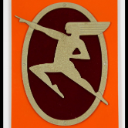1161
4
03-18-2019 10:05 AMOccasional Contributor

Hello all,

I have a map with two polygon layers and a point layer. One polygon layer is isochrones representing how far someone can go on public transit using current schedules. The second polygon layer is isochrones representing how far someone can go with increased frequency. The point layer contains points of interest. Some of these points can be reached by both polygons, some are only reached by one polygon, and some are not reached by either polygon. I'm trying to build an expression that I can use in the pop-up that will tell the viewer if that point can be reached using the current schedule, the increased frequency schedule, both, or neither. Below is the expression:

``````var current = FeatureSetByName(\$map,"Jane with Current Schedules")
var increased = FeatureSetByName(\$map,"Jane with High Frequency")

var Jane_current = Intersects(\$feature,current)
var Jane_increased = Intersects(\$feature,increased)
var both_Janes = Iif(Jane_current == Jane_increased,'true','false')

When(both_Janes == 'true','This location can be reached by Jane using the current and increased frequency schedules.'
Jane_current == true,'This location can be reached by Jane using the current public transportation schedules.',
Jane_increased == true,'This location can be reached by Jane using the increased frequency schedule.','This location cannot be reached by public transportation.')
‍‍‍‍‍‍‍‍‍‍‍‍‍‍‍‍‍‍‍‍‍‍‍‍‍‍‍‍‍‍‍‍‍‍‍‍‍‍‍‍‍‍‍‍‍‍‍‍‍‍‍‍‍‍‍‍‍‍‍‍‍‍‍‍‍``````

When I try to run this, I get the following error: Parse Error:Line 9: Unexpected identifier

If I put a Count function around the intersects, it returns the correct count, so I'm confused why I'm getting an error on Line 9 when I call Jane_current in the When function.

Any help would be appreciated.

Miguel

Miguel Fernandez
Tags (2)
1 Solution

Accepted SolutionsbyMVP Honored Contributor

You're missing a comma at the end of this line

``````When(both_Janes == 'true','This location can be reached by Jane using the current and increased frequency schedules.'‍
``````
4 RepliesbyMVP Honored Contributor

You're missing a comma at the end of this line

``````When(both_Janes == 'true','This location can be reached by Jane using the current and increased frequency schedules.'‍
``````Occasional Contributor

Ken,

Thank you so much for spotting that!! I can't tell you how many times I went through this.

Miguel

Miguel FernandezOccasional Contributor

I will say that my expression does not produce the desired results. All points say that "This location cannot be reached by public transportation." So if you come across this post and know what might be causing that, feel free to let me know!

Miguel FernandezbyMVP Honored Contributor

This works properly in the Arcade playground, changing the various permutations of true and false

``````var Jane_current = true;
var Jane_increased = true;

var both_Janes = Iif(Jane_current && Jane_increased,true,false)

When(both_Janes == true,'This location can be reached by Jane using the current and increased frequency schedules.',
Jane_current == true,'This location can be reached by Jane using the current public transportation schedules.',
Jane_increased == true,'This location can be reached by Jane using the increased frequency schedule.','This location cannot be reached by public transportation.')‍‍‍‍‍‍‍‍‍‍‍‍‍‍‍‍``````